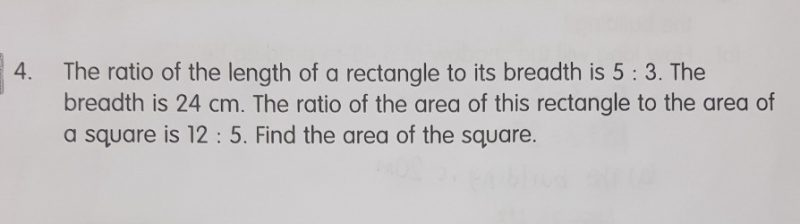# QuestionRatio of Length to Breadth = 5 : 3,

so if Breadth is 24 cm,

Length = 5 x 24/3  cm = 5 x 8 cm = 40 cm.

Now ratio of area of this rectangle to a square = 12 : 5, and

Area of this rectangle is Length x Breadth = 40 x 24 sq cm = 960 sq cm,

So if 12 units (from Ratio 12 : 5 ) is 960 sq cm, then 1 unit is 960/12 sq cm  = 80 sq cm.

Therefore, 5 units (representing the square from the above Ratio) = 80 x 5 sq cm = 400 sq cm.

Area of the square is 400 sq cm.

0 Replies 0 Likes